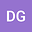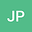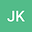On the spatially inhomogeneous particle coagulation-condensation model with singularity
•••Jitendra Kumar
Indian Institute of Technology Kharagpur
Author Profile## Abstract

The spatially inhomogeneous coagulation-condensation process is an interesting topic of study as the phenomenon’s mathematical aspects mostly undiscovered and has multitudinous empirical applications. In this present exposition, we exhibit the existence of a continuous solution for the corresponding model with the following \emph{singular} type coagulation kernel: $K(x,y)~\le~\frac{\left( x + y\right)^\theta}{\left(xy\right)^\mu}, ~~\text{for} ~x, y \in (0,\infty), \text{where}~ \mu \in \left[0,\tfrac{1}{2}\right] \text{ and } ~\theta \in [0, 1].$ The above-mentioned form of the coagulation kernel includes several practical-oriented kernels. Finally, uniqueness of the solution is also investigated.